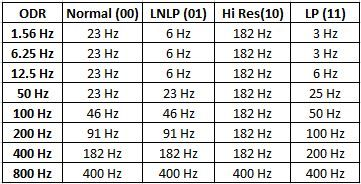## MMA8451Q Low-pass cutoff frequency

cancel
Showing results for
Did you mean:
SOLVED

## MMA8451Q Low-pass cutoff frequency

967 ViewsContributor I

The MMA8451q data sheet and various application notes all make mention of the output data being low-pass filtered by default (see, for example, AN4076 section 6.1 - http://cache.freescale.com/files/sensors/doc/app_note/AN4076.pdf  ​).

However, no specifics are given on the cutoff frequency of the low-pass filter, should it be left enabled (by neglecting to enable the high-pass filter).

Is the cutoff frequency tied to (or equal to) the Output Data Rate?

Any help is appreciated.

Labels (1)
• ### Accelerometers

1 Solution
280 ViewsNXP TechSupport

Hi Samuel,

The low-pass digital filter is a simple 1st order decimation filter. Its cut-off frequency depends on ODR and MODS settings. If you look at table 67 of the MMA8451Q datasheet, you will find a column "OS Ratio" for all possible ODR/MODS combinations. This table allows you to calculate the ADC frequency, following the formula:

ADC freq = OS Ratio * ODR

The low-pass filter does a decimation by either 2 or 4, according to the following rule and the cut-off frequency depends on the decimation rate:

OS Ratio = 2:  decimation by 2, cut-off freq = 0.25 * ADC freq

OS Ratio >= 4: decimation by 4, cut-off freq = 0.114 * ADC freq

The following table summarizes it well.Regards,

Tomas

281 ViewsNXP TechSupport

Hi Samuel,

The low-pass digital filter is a simple 1st order decimation filter. Its cut-off frequency depends on ODR and MODS settings. If you look at table 67 of the MMA8451Q datasheet, you will find a column "OS Ratio" for all possible ODR/MODS combinations. This table allows you to calculate the ADC frequency, following the formula:

ADC freq = OS Ratio * ODR

The low-pass filter does a decimation by either 2 or 4, according to the following rule and the cut-off frequency depends on the decimation rate:

OS Ratio = 2:  decimation by 2, cut-off freq = 0.25 * ADC freq

OS Ratio >= 4: decimation by 4, cut-off freq = 0.114 * ADC freq

The following table summarizes it well.Regards,

Tomas